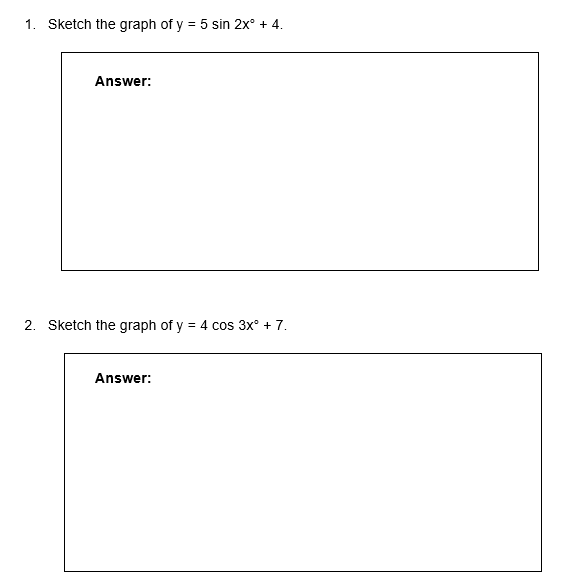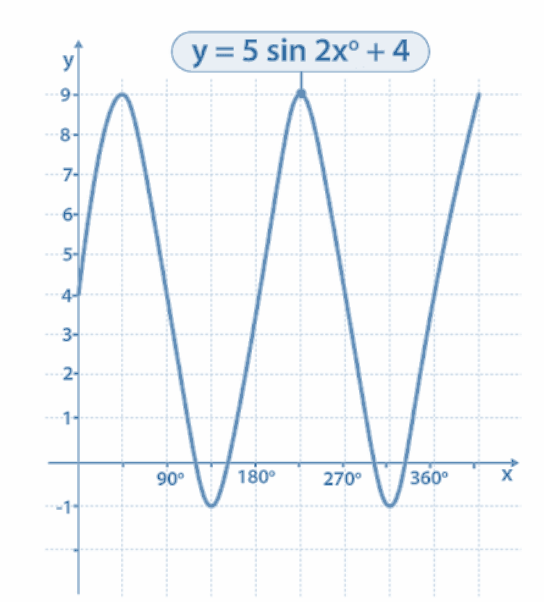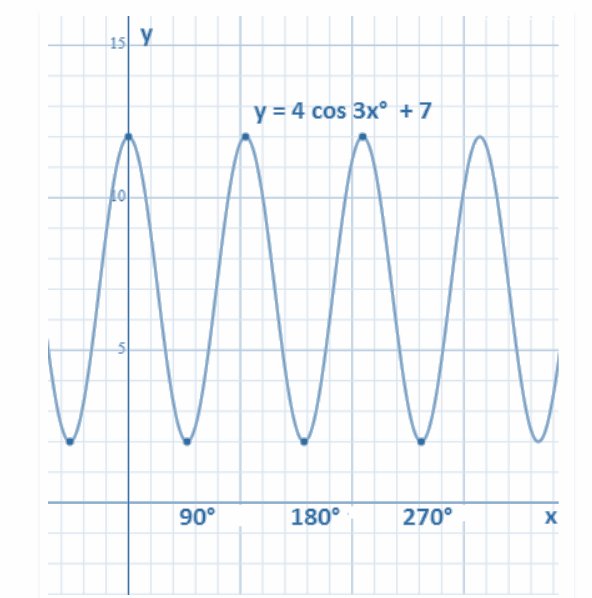# Graphing Trigonometric Functions Worksheet (with answer key + PDF)

Trigonometry is used to establish directions like the north, south, east, and west. It also tells you which direction to point the compass in order to travel straight ahead. To locate a specific location, it is used in navigation. It is also employed to calculate the separation between a point in the sea and the shore.

## What is the “Graphing Trigonometric Functions Worksheet (with answer key + PDF)”?

This worksheet will explore some of the graphing trigonometric functions, which are the sine, cosine, and tangent. Here we will discuss how these trigonometric functions with the graph.

## What are graphing trigonometric functions?

In these trigonometry graphs, the y-axis represents the value of the function at each given angle, and the x-axis represents the angle values in radians.

This worksheet will help the student to understand the graphing trigonometric functions. Here we provided some information about this particular topic.

Instructions on how to use the “So Graphing Trigonometric Functions Worksheet (with answer key + PDF).”

Study the concept and examples given and try to solve the given exercises below.

## Conclusion

They are employed in the modeling of numerous different mechanical and natural phenomena (populations, waves, engines, acoustics, electronics, UV intensity, growth of plants and animals, etc.). The trigonometric graphs in this chapter are periodic, which means that after a predetermined interval, the shape repeats exactly.

If you have any inquiries or feedback, please let us know.

## Graphing Trigonometric Functions Worksheet (with answer key + PDF)

The three crucial trigonometry ratios on which functions are defined are sine, cosine, and tangent. The graphs for the three trigonometric functions sin, cos, and tan are shown below. In these trigonometry graphs, the y-axis represents the value of the function at each given angle, and the x-axis represents the angle values in radians.

Sin Graph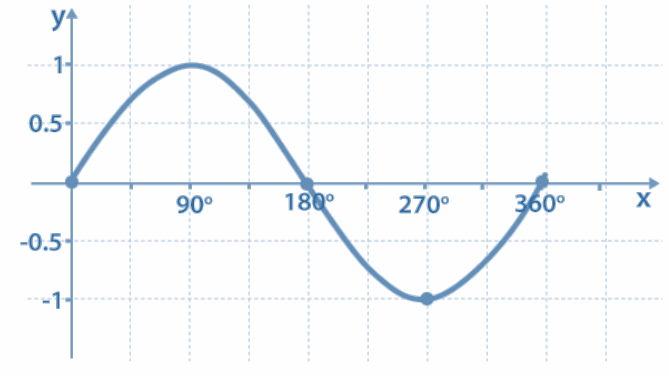• y = sin x
• The roots or zeros of y = sin x are at the multiples of π
• The sin graph passes the x-axis as sin x = 0 there
• The period of the sine function is
• The height of the curve at each point is equal to the line value of the sine

Max value of Graph: 1 at π/2

Min value of the graph: -1 at (3π/2)

Cos Graph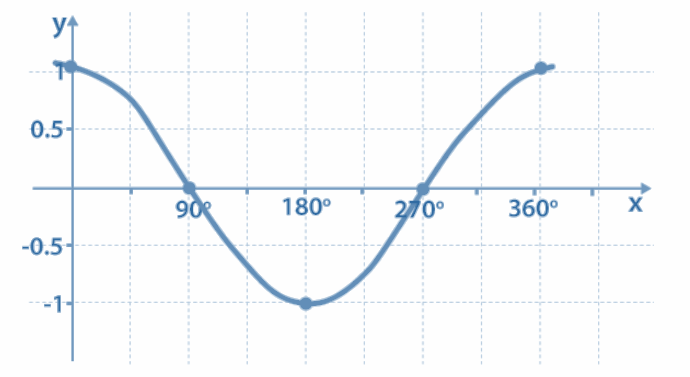• y = cos x
• sin (x + π/2) = cos x
• y = cos x graph is the graph we get after shifting y = sin x to π/2 units to the left
• The period of the cosine function is

Max value of Graph ; 1 at 0, 4π

Min value of the graph: -1 at 2π

Tan Graph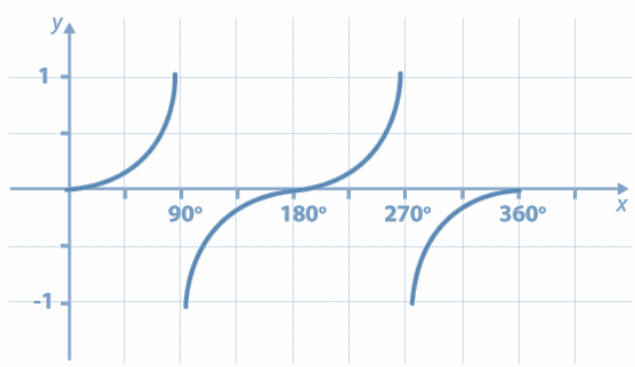• y = tan x
• The tangent graph has an undefined amplitude as the curve tends to infinity
• It also has a period of 180°, i.e., π

Amplitude

• It is the absolute value of any number multiplied by it on the trigonometric function.
• The height from the center line to the peak (or trough) is called amplitude.
• You can also measure the height from the highest to lowest points and then divide it by 2.
• It basically tells how tall or short the curve is.
• Also, notice that the function is in the usual orientation or upside down depending on the minus or plus sign of the amplitude value.

Period

• The Period goes from any point (one peak) to the next matching point.
• The graphical representation of the period and amplitude of a function is given below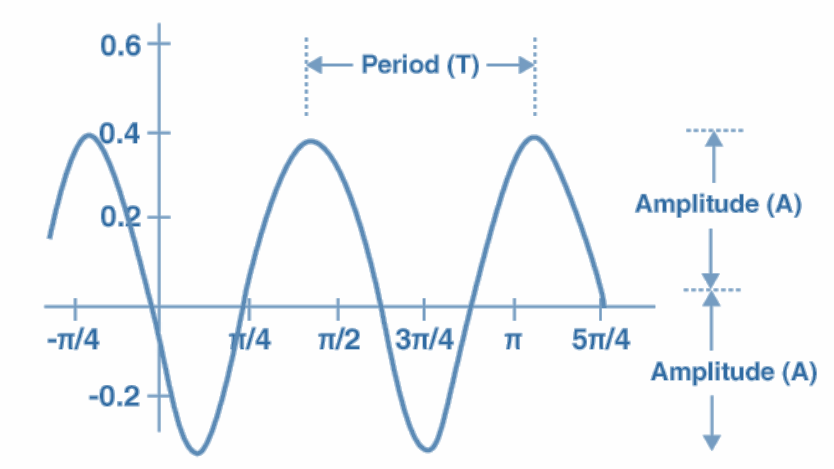## Worksheet

Graphing Trig Functions Worksheet.